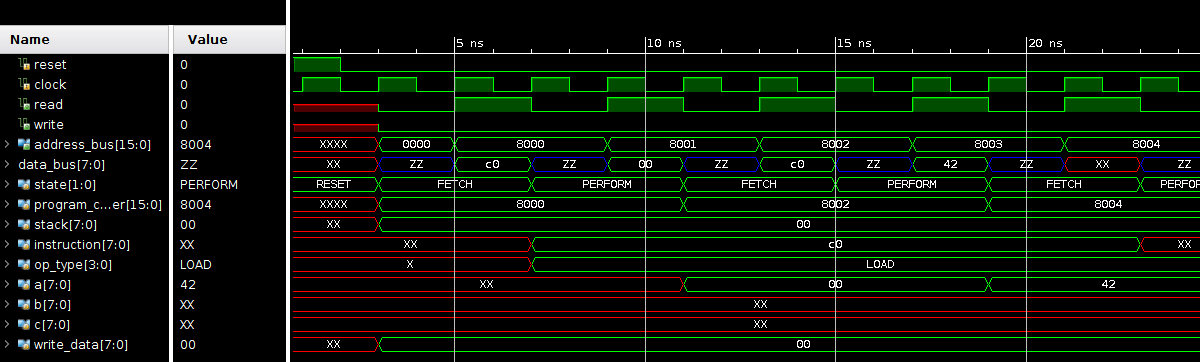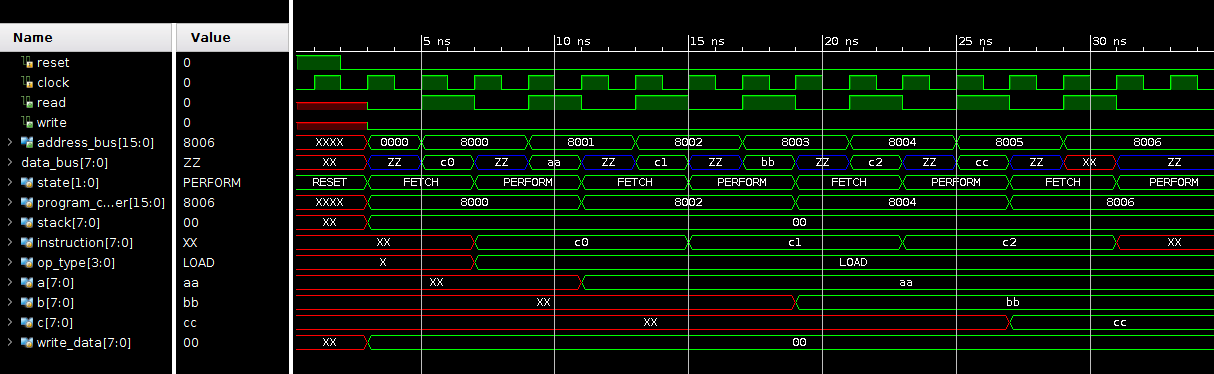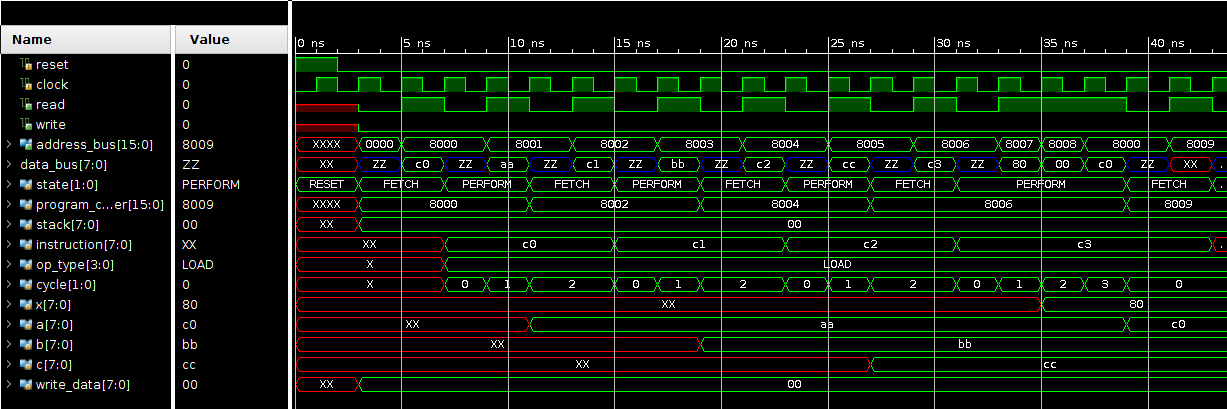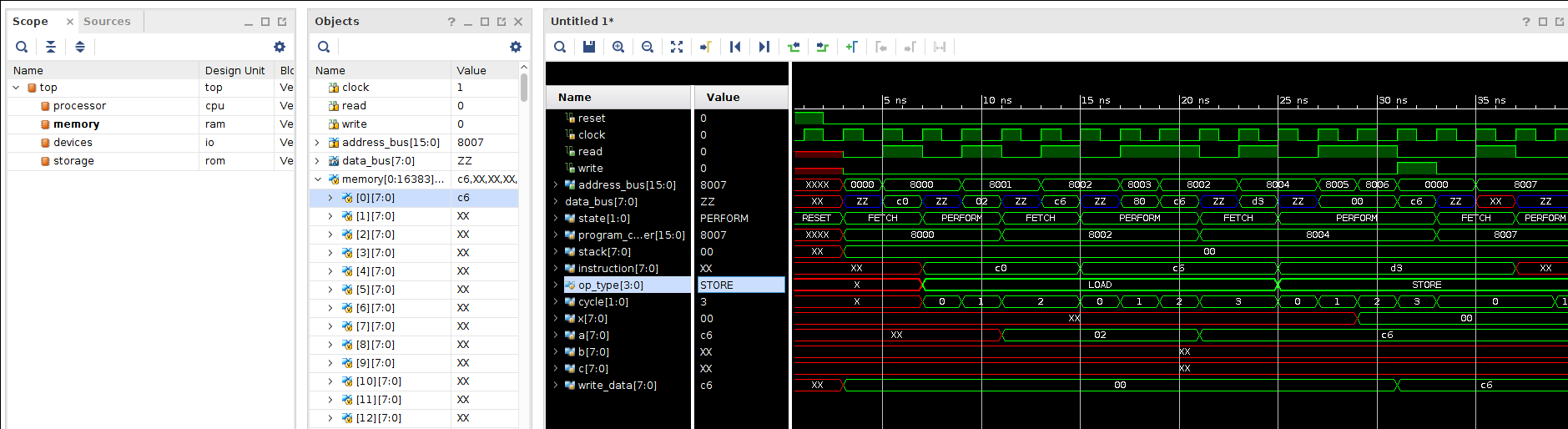# Beginning Logic Design – Part 11

Hello and welcome to Part 11 of my Beginning Logic Design series. In this episode, I will continue implementing the CPU I planned and stared in the previous post.

The first CPU operations I’d like to have working are going to be my LOAD and STORE type instructions, as these provide the basic reading and writing operations to interact with my system bus. This design will not be very efficient nor the most clever implementation, but it will work!

# The LOAD Instruction

I want each of my registers to have the same `LOAD` capabilities, in contrast to the 6502 instruction set which has 8 types of `LOAD` instructions for `A` and 5 for `X` and `Y`.

I have 5 types of load instruction in mind:

• Load register with next byte in program code (Immediate Load)
• Load register using next two bytes of program code as a memory address. (Memory Load)
• Load register using next byte as the upper 4 bits of a memory address, and the `A` register as the lower 4 bits (A indexed load)
• Load register using next byte as the upper 4 bits of a memory address, and the `B` register as the lower 4 bits (B indexed load)
• Load register using next byte as the upper 4 bits of a memory address, and the `C` register as the lower 4 bits (C indexed load)

With my 3 registers and these 5 different types of load instructions, this consumes 15 of the 16 possible LOAD instructions.

1. 0 - Immediate Load A
2. 1 - Immediate Load B
3. 2 - Immediate Load C
4. 3 - Memory Load A
5. 4 - Memory Load B
6. 5 - Nemory Load C
7. 6 - A Index Load A
8. 7 - A Index Load B
9. 8 - A Index Load C
10. 9 - B Index Load A
11. a - B Index Load B
12. b - B Index Load C
13. c - C Index Load A
14. d - C Index Load B
15. e - C Index Load C
16. f - undefined

With a rough plan, I’m ready to start implementing! My first goal is to just get the Immediate Load A instruction to work. I’ll write a small program that should load A with `00` then load it with `42`.

1. c0 00
2. c0 42

Now I’ll start building out what will end up being a huge tree of `case` statements implementing my various operations. This isn’t the most elegant way to organize the code, but it’s simple and it will work for a start.

1. PERFORM: begin
2. case (op_type)
4. case (instruction[3:0])
5. 0: begin
6. if (!read) begin
7. read <= 1;
8. address_bus <= program_counter + 1;
9. end else begin
10. read <= 0;
11. a <= data_bus;
12. program_counter += 2;
13. state <= FETCH;
14. end
15. end
16. endcase
17. end
18. endcase
19. end

Similar to the fetch state this will be a two cycle operation. First the CPU starts a memory read request for the next byte in program code, on the next cycle the result is stored into the program counter. The `program_counter` then gets incremented to the next address after the opcode and its parameter.

Testing this bit of code in simulation verifies it works as intended!From here we can use our copy-pasta skills to do the same for the immediate load operations for the `B` and `C` registers.

2. case (instruction[3:0])
3. 0: begin
4. if (!read) begin
5. read <= 1;
6. address_bus <= program_counter + 1;
7. end else begin
8. read <= 0;
9. a <= data_bus;
10. program_counter += 2;
11. state <= FETCH;
12. end
13. end
14. 1: begin
15. if (!read) begin
16. read <= 1;
17. address_bus <= program_counter + 1;
18. end else begin
19. read <= 0;
20. b <= data_bus;
21. program_counter += 2;
22. state <= FETCH;
23. end
24. end
25. 2: begin
26. if (!read) begin
27. read <= 1;
28. address_bus <= program_counter + 1;
29. end else begin
30. read <= 0;
31. c <= data_bus;
32. program_counter += 2;
33. state <= FETCH;
34. end
35. end
36. endcase
37. end

I’ll write a new program to test this out:

1. c0 aa
2. c1 bb
3. c2 cc

Stepping through these opcodes, I should end up with the `A` register set to `aa`, `B` to `bb` and `C` to `cc`.Woohoo! These load commands work and were not too difficult to implement. At this point I’m feeling pretty excited about my first CPU design.

For my next trick, I will implement my memory load operations. These will fetch a memory address after the current instruction and set the register to the number at that location. This operation is going to take more than two CPU cycles. With this in mind, I’m going to add a new internal variable `logic [1:0] cycle;` to track each CPU cycle. In my `FETCH`, state, I will set `cycle` to `0` as I transition to the `PERFORM` state so that all instructions can use this same variable. After the main `case` statement within `PERFORM`, I’ll add `cycle++;` to increment `cycle` every clock cycle.

Next, before I implement my memory load operations, I’ll modify the immediate load implementation to follow this model for consistency.

2. case (instruction[3:0])
3. 0: begin
4. case (cycle)
5. 0: begin
6. read <= 1;
7. address_bus <= program_counter + 1;
8. end
9. 1: begin
10. read <= 0;
11. a <= data_bus;
12. program_counter += 2;
13. state <= FETCH;
14. end
15. endcase
16. end
17. ...

Now for the memory load! It will start off identical to the immediate load by reading the next byte in code.

1. // Memory load A
2. 3: begin
3. case (cycle)
4. 0: begin
5. read <= 1;
6. address_bus <= program_counter + 1;
7. end
8. endcase
9. end

On the next cycle I’ll have the most significant address byte returned via the data bus and I’ll need another register to store it. I’ll add `logic [7:0] x;` near my other CPU internal registers and request the next byte.

1. 1: begin
2. x <= data_bus;
3. address_bus <= program_counter + 2;
4. end

On the 3rd cycle, I’ll have the lower address byte and can concatenate it with the `x` register to read that memory address.

1. 2: begin
2. address_bus <= {x,data_bus};
3. end

Finally, on the last cycle of the operation, I will have the value from the specified memory location on the `data_bus`. I can store that value, increment the `program_counter` by the total length of the instruction, clear the `read` signal and transition back into `FETCH`.

1. 3: begin
2. program_counter += 3;
3. read <= 0;
4. a <= data_bus;
5. state <= FETCH;
6. end

Now to test it! I’ll extend my previous program to include this new operation. It’ll load the first byte of the program into A.Alright! It does successfully pull the memory address and uses it to load the value at that address into the register. With some more copy paste I can replicate this for the `B` and `C` registers.

## Offset Memory Load

With the basic memory load operation figured out, the offset memory load is a small modification. I only need to read the most significant address byte then I can concatenate that with the appropriate register to read the desired offset address.

1. // A offset load A
2. 6: begin
3. case (cycle)
4. 0: begin
5. read <= 1;
6. address_bus <= program_counter + 1;
7. end
8. 1: begin
9. address_bus <= {data_bus, a};
10. end
11. 2: begin
12. program_counter += 2;
13. read <= 0;
14. a <= data_bus;
15. state <= FETCH;
16. end
17. endcase
18. end

As before I can duplicate this for the various permutations of the load command. I validated this in the simulator as well and it looks to work just as intended.

# Store Operations

The `STORE` operations are nearly identical to the load operations, though there are not Immediate Store instructions. Because the operations are so similar I will actually even use the same numbers for the lower 4 operation bits to indicate the types of operation.

1. 0 - undefined
2. 1 - undefined
3. 2 - undefined
4. 3 - Memory Store A
5. 4 - Memory Store B
6. 5 - Nemory Store C
7. 6 - A Index Store A
8. 7 - A Index Store B
9. 8 - A Index Store C
10. 9 - B Index Store A
11. a - B Index Store B
12. b - B Index Store C
13. c - C Index Store A
14. d - C Index Store B
15. e - C Index Store C
16. f - undefined

I’ll first implement the Memory Store A operation. It starts off pretty similar to the load, as it needs to begin by reading the memory address from the program code.

1. 0: begin
2. read <= 1;
3. address_bus <= program_counter + 1;
4. end
5. 1: begin
6. x <= data_bus;
7. address_bus <= program_counter + 2;
8. end

On the next cycle, I’ll have the full address and can stop reading to start writing `A` to the `data_bus`. On the last cycle I’ll increment the program counter and return to `FETCH` to grab the next bit of code.

1. 2: begin
2. address_bus <= {x,data_bus};
3. read <= 0;
4. write <= 1;
5. write_data <= a;
6. end
7. 3: begin
8. program_counter += 3;
9. write <= 0;
10. state <= FETCH;
11. end

Easy enough! I’ll extend my last program to end with an operation to write A to the first byte of my RAM.

1. c0 02
2. c6 80
3. d3 00 00

Via simulation I can confirm it’s stashing the `A` register into the first byte of RAM.As before we can use this as the basis for the memory store calls for the `B` and `C` registers.

## Offset Store Operations

As the offset load was a small variation on memory load, the same will be true for offset store. With some small modifications to the regular memory store call, the offset store is easily implemented.

1. // A offset store A
2. 6: begin
3. case (cycle)
4. 0: begin
5. read <= 1;
6. address_bus <= program_counter + 1;
7. end
8. 1: begin
9. address_bus <= {data_bus, a};
10. read <= 0;
11. write <= 1;
12. write_data <= a;
13. end
14. 2: begin
15. program_counter += 2;
16. write <= 0;
17. state <= FETCH;
18. end
19. endcase
20. end

With the STORE and LOAD operations implemented I will call it a wrap for this post. As always I would love any feedback or questions you may have. Keep tinkering!

Posted on Categories Digital Logic Design